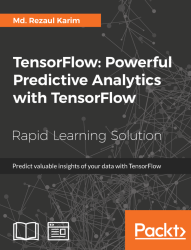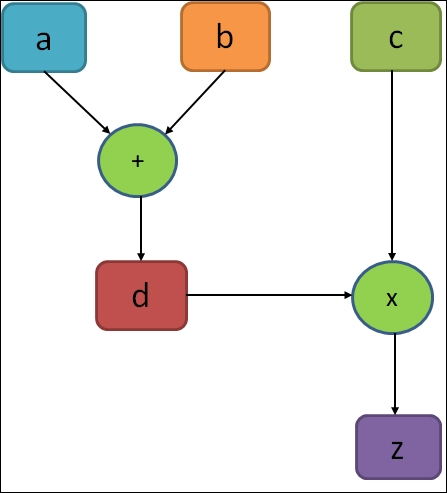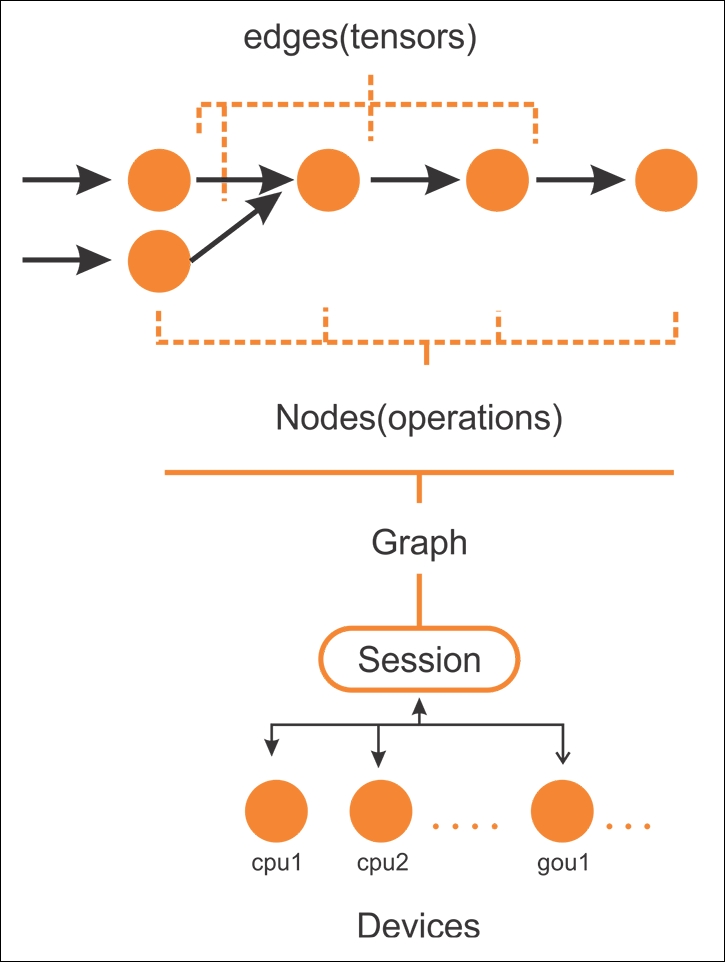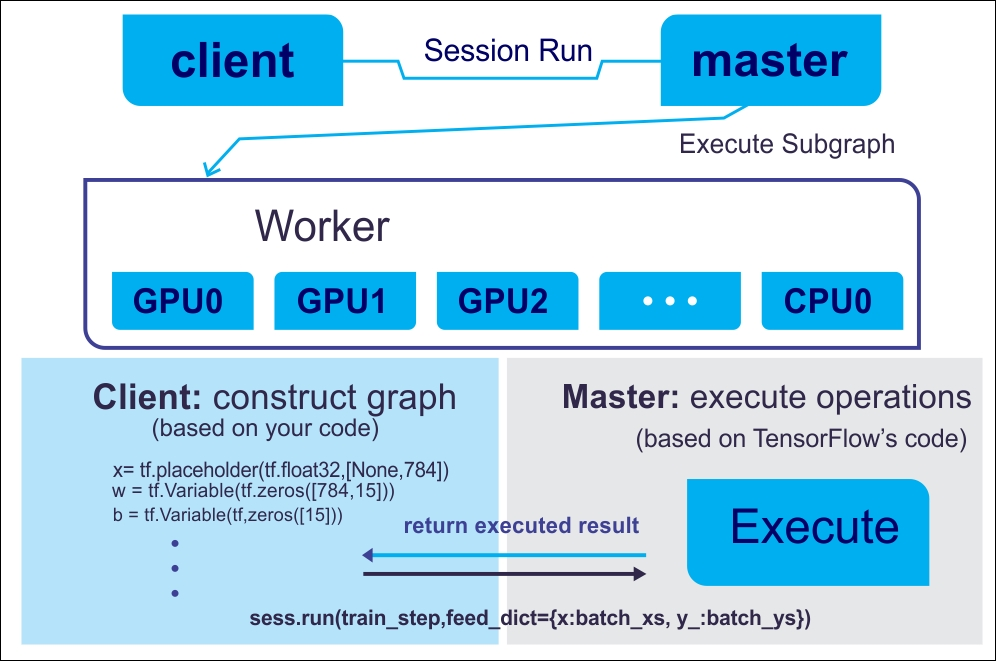•#### TensorFlow: Powerful Predictive Analytics with TensorFlow#### Overview of this book

Predictive analytics discovers hidden patterns from structured and unstructured data for automated decision making in business intelligence. Predictive decisions are becoming a huge trend worldwide, catering to wide industry sectors by predicting which decisions are more likely to give maximum results. TensorFlow, Google’s brainchild, is immensely popular and extensively used for predictive analysis. This book is a quick learning guide on all the three types of machine learning, that is, supervised, unsupervised, and reinforcement learning with TensorFlow. This book will teach you predictive analytics for high-dimensional and sequence data. In particular, you will learn the linear regression model for regression analysis. You will also learn how to use regression for predicting continuous values. You will learn supervised learning algorithms for predictive analytics. You will explore unsupervised learning and clustering using K-meansYou will then learn how to predict neighborhoods using K-means, and then, see another example of clustering audio clips based on their audio features. This book is ideal for developers, data analysts, machine learning practitioners, and deep learning enthusiasts who want to build powerful, robust, and accurate predictive models with the power of TensorFlow. This book is embedded with useful assessments that will help you revise the concepts you have learned in this book. This book is repurposed for this specific learning experience from material from Packt's Predictive Analytics with TensorFlow by Md. Rezaul Karim.
TensorFlow: Powerful Predictive Analytics with TensorFlowCreditsPrefaceFree Chapter
From Data to Decisions – Getting Started with TensorFlowPutting Data in Place – Supervised Learning for Predictive AnalyticsClustering Your Data – Unsupervised Learning for Predictive AnalyticsUsing Reinforcement Learning for Predictive Analytics## TensorFlow Computational Graph

When thinking of execution of a TensorFlow program we should be familiar with a graph creation and a session execution. Basically the first one is for building the model and the second one is for feeding the data in and getting the results. An interesting thing is that TensorFlow does each and everything on the C++ engine, which means even a little multiplication or addition is not executed on Python but Python is just a wrapper. Fundamentally, TensorFlow C++ engine consists of following two things:

• Efficient implementations for operations like convolution, max pool, sigmoid, and so on.

• Derivatives of forwarding mode operation.

When we/you're performing a little complex operation with TensorFlow, for example training a linear regression, TensorFlow internally represents its computation using a dataflow graph. The graph is called a computational graph, which is a directed graph consisting of the following:

• A set of nodes, each one representing an operation

• A set of directed arcs, each one representing the data on which the operations are performed.

TensorFlow has two types of edges:

• Normal: They carry the data structures between the nodes. The output of one operation from one node, becomes input for another operation. The edge connecting two nodes carries the values.

• Special: This edge doesn't carry values, but only represents a control dependency between two nodes, say X and Y. It means that the node Y will be executed only if the operation in X is executed already, but before the relationship between operations on the data.

The TensorFlow implementation defines control dependencies to enforce orderings between otherwise independent operations as a way of controlling the peak memory usage.

A computational graph is basically like a dataflow graph. Figure 5 shows a computational graph for a simple computation like z=d×c=(a+b) ×c:Figure 5: A very simple execution graph that computes a simple equation

In the preceding figure, the circles in the graph indicate the operations, while rectangles indicate a data computational graph. As stated earlier, a TensorFlow graph contains the following:

• A set of tf.Operation objects: This is used to represent units of computation to be performed

• A tf.Tensor object: This is used to represent units of data that control the dataflow between operations

Using TensorFlow, it is also possible to perform a deferred execution. To give an idea, once you have composed a highly compositional expression during the building phase of the computational graph, you can still evaluate them in the running session phase. Technically saying TensorFlow schedules the job and executes on time in an efficient manner. For example, parallel execution of independent parts of the code using the GPU is shown in figure 6.Figure 6: Edges and nodes in TensorFlow graph to be executed under a session on devices such as CPUs or GPUs

After a computational graph is created, TensorFlow needs to have an active session to be executed by multiple CPUs (and GPUs if available) in a distributed way. In general, you really don't need to specify whether to use a CPU or a GPU explicitly, since TensorFlow can choose and use which one is to be used. By default, a GPU will be picked for as many operations as possible; otherwise, a CPU will be used. So in a broad view, here are the main components of TensorFlow:

• Variables: Used to contain values for the weights and bias between TensorFlow sessions.

• Tensors: A set of values that pass in between nodes.

• Placeholders: Is used to send data between the program and the TensorFlow graph.

• Session: When a session is started, TensorFlow automatically calculates gradients for all the operations in the graph and use them in a chain rule. In fact, a session is invoked when the graph is to be executed.

Don't worry much, each of the preceding components will be discussed in later sections. Technically saying, the program you will be writing can be considered as a client. The client is then used to create the execution graph in C/C++ or Python symbolically, and then your code can ask TensorFlow to execute this graph. See details in the following figure:Figure 7: Using a client–master architecture for executing TensorFlow graph

A computational graph helps to distribute the work load across multiple computing nodes having a CPU or a GPU. This way, a neural network can further be equaled to a composite function where each layer (input, hidden or output layer) can be represented as a function. Now to understand the operations performed on the tensors, knowing a good workaround about TensorFlow programming model is a mandate. The next section explains the role of the computational graph to implement a neural network.